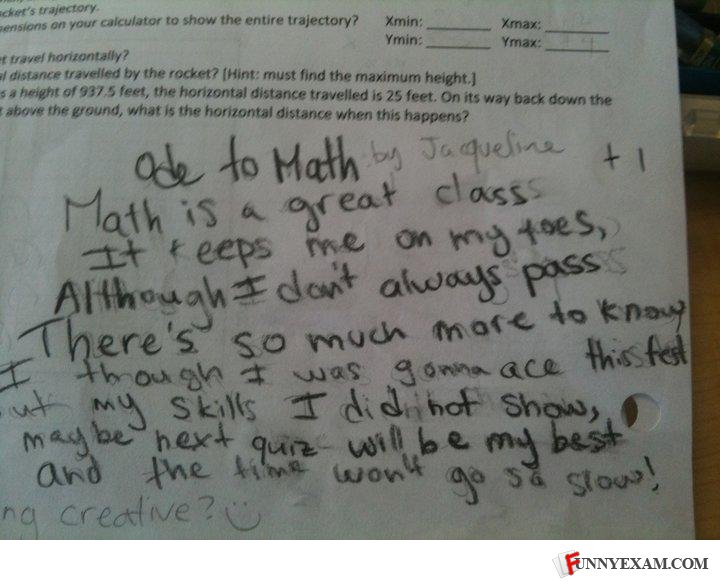1.2 by 1,024 huffers# Math is a wonderful thing. Yes, math is a really cool thing. So get off your ath, let's do some math. Math, math, math, math, math.

## Other's Responses

• Math is a wonderful thing. Yes, math is a really cool thing. So get off your ath, let's do some math. Math, math, math, math, math.

• Isn't this physics?